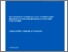< back to main site

## Development of consistent local thermodynamic relations for non-equilibrium multi-component fluid systems

McCartney, L N; Dickinson, E J (2021) Development of consistent local thermodynamic relations for non-equilibrium multi-component fluid systems. NPL Report. MAT 98Preview
Text (NPL Report MAT 98)
MAT98.pdf - Published Version

## Abstract

This report addresses the issue of formulating thermodynamic equations of state for multi-component chemical systems that can be consistently applied locally (on a volumetric, molar or specific basis) and so can form the thermodynamic basis of a theory for non-equilibrium transport in non-uniform multi-component reacting fluids.

The development of local equations of state, for application in non-uniform multi-component fluid systems, requires the introduction of localised composition variables, such as mole or mass fractions, which are not independent. Robust, unambiguous methods for the mathematical handling of such dependencies are developed, while maintaining close contact with equivalent global thermodynamic relations encountered in uniform systems. While four candidate thermodynamic functions can be the basis of a local equation of state, associated respectively with internal energy, Helmholtz energy, Gibbs energy and enthalpy, the most practically useful is based on the molar Gibbs energy for which the function depends only on intensive state variables (temperature, pressure and composition).

For global thermodynamic systems applicable to multi-component uniform systems having n species in equilibrium, equations of state in the form of Gibbs energy functions (homogeneous of order unity with respect to composition variables) are often known at the outset, enabling the development of corresponding local relations required for simulation purposes. Localisation based on the molar Gibbs energy, using the total number of moles of all species N, leads to state variables for composition which are not independent, i.e. the set of n mole fractions {xi}. By comparison, localisation with respect to a fixed total number of moles of all species N* rather than N, leads to the concept of pseudo-mole fractions {xi*}. The set of n variables are independent, but tend to the dependent set of mole fractions {xi} when N* -> N. The molar Gibbs energy function resulting from the latter localisation procedure, expressed in terms of pseudo-mole fractions, can be used to generate chemical potentials, and other thermodynamic molar quantities, by using single partial derivatives in a manner analogous to the well-defined approach used for uniform global systems.

Great care is needed when calculating chemical potentials from general molar Gibbs energy functions sourced from literature or experimental data, as it is highly likely that the derived molar Gibbs energy function will lack the homogeneity needed for the use of the single partial derivative formula for chemical potential. A new expression is derived to compute chemical potentials when using a non-homogeneous molar Gibbs energy functions, and a method is presented to construct the homogeneous molar Gibbs energy function of a particular system from any non-homogeneous molar Gibbs energy function. This leads self-consistently to correct general formulae for chemical potentials expressed in terms of intensive state variables, and hence for all partial molar properties for use in local thermodynamic relations.

It is observed that an expression for partial pressure of any species of the system has been proposed that applies to non-ideal multi-component systems, and which reduces to the commonly used expression that has been established only for ideal systems. Alternative thermodynamic formulations based on specific rather than molar quantities, and a molar formulation based on n – 1 independent mole fractions, are briefly considered.

Item Type: Report/Guide (NPL Report) MAT 98 Advanced Materials > Thermodynamics Electromagnetic & Electrochemical Technologies 10.47120/npl.MAT98 12 Jul 2021 14:56 http://eprintspublications.npl.co.uk/id/eprint/9179View Item